1 Jun 2023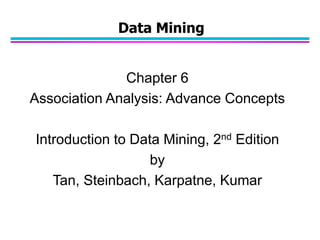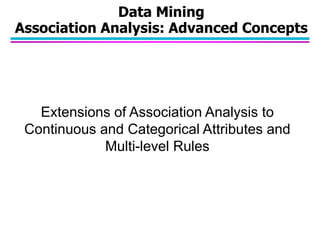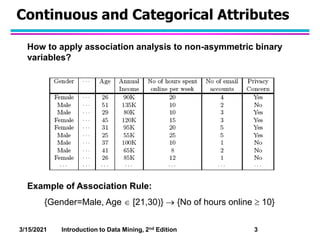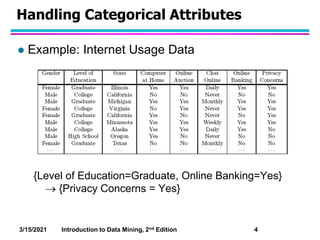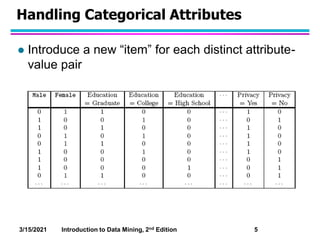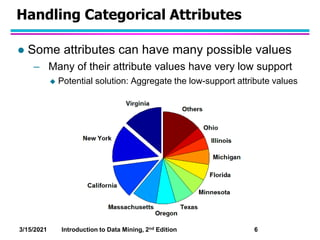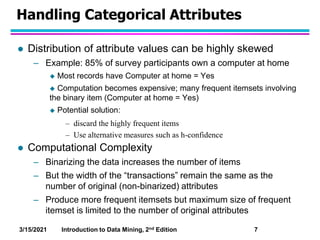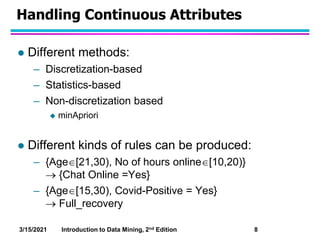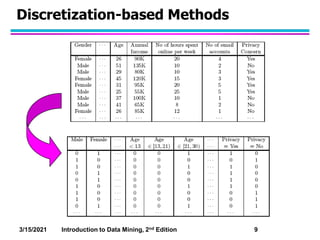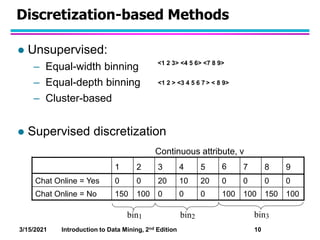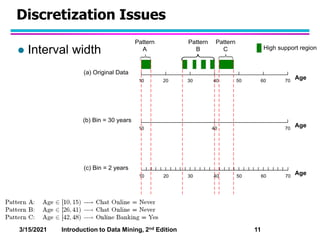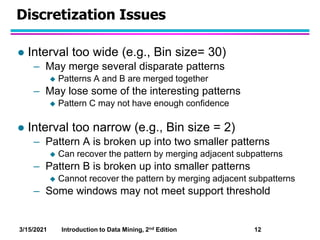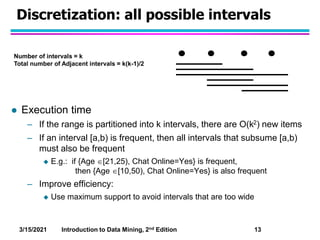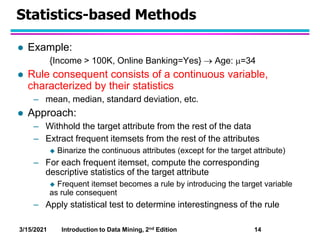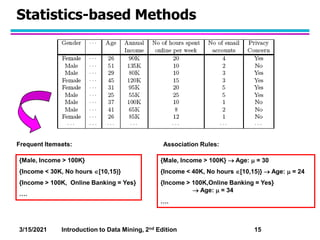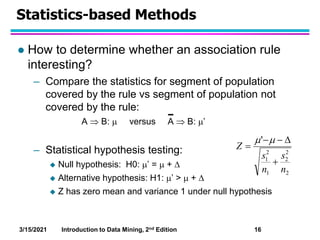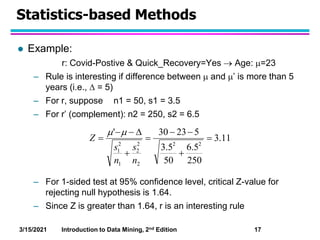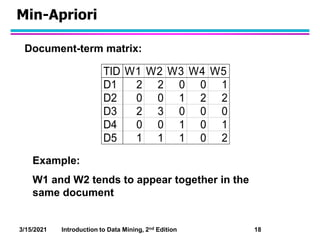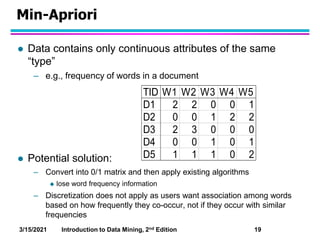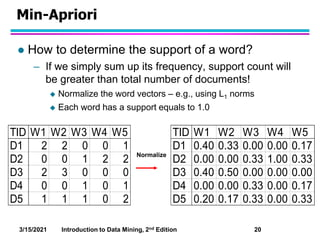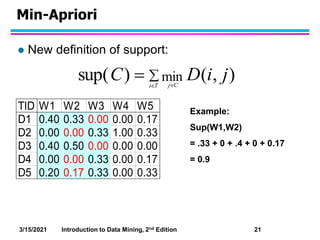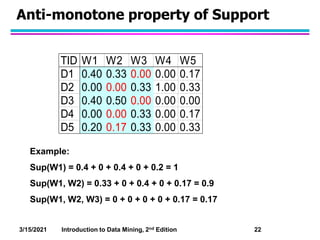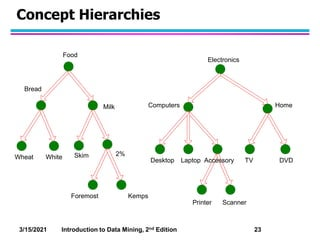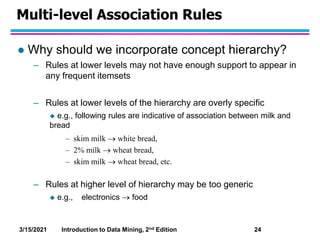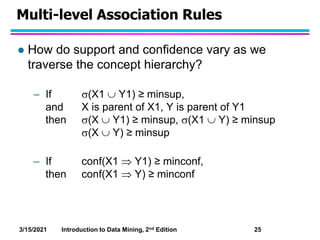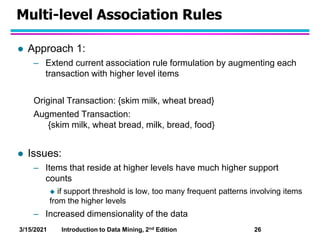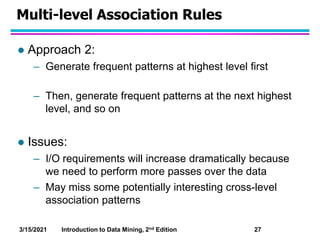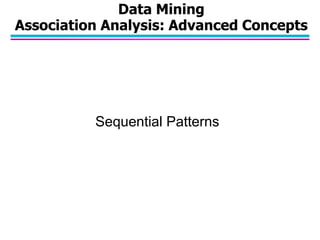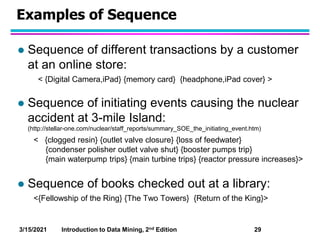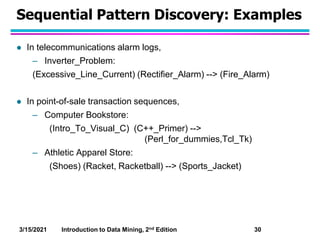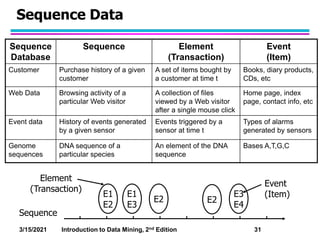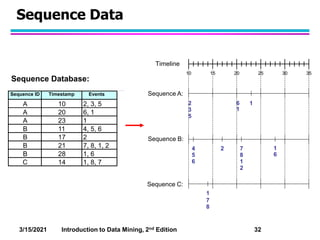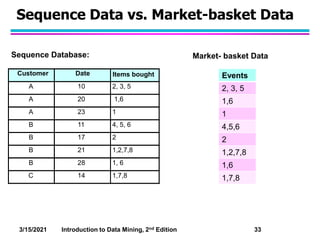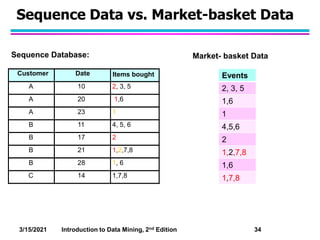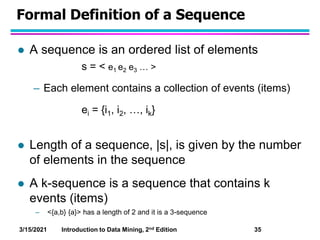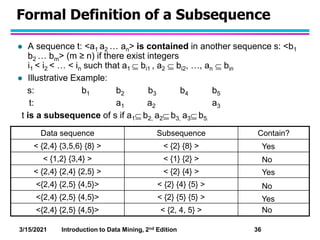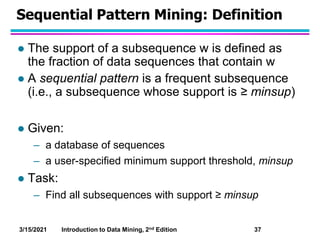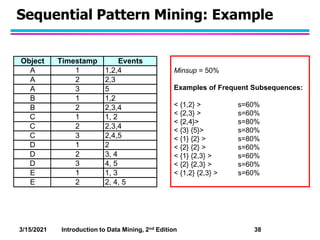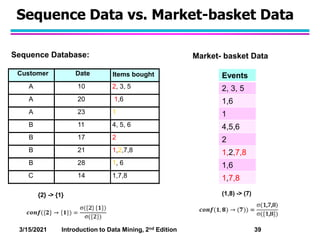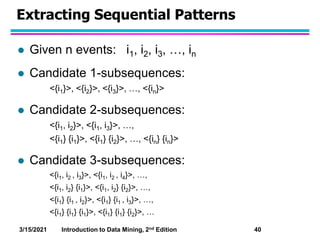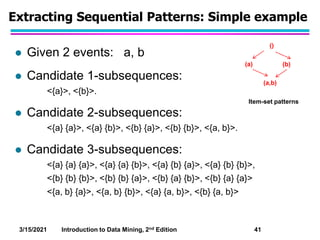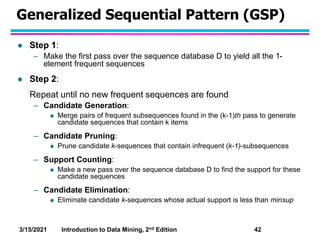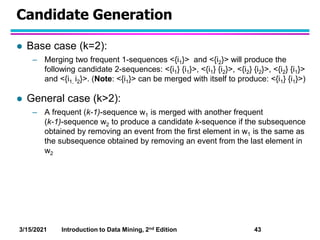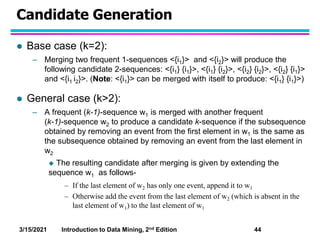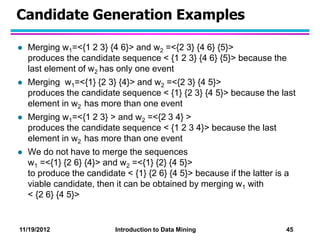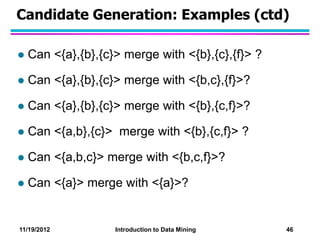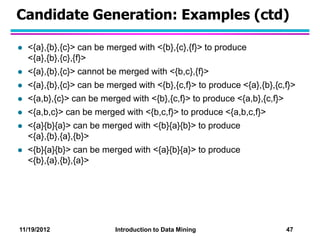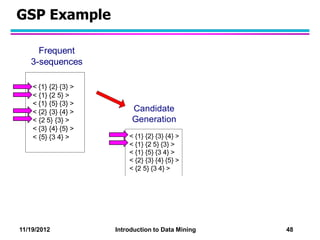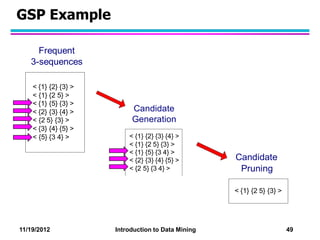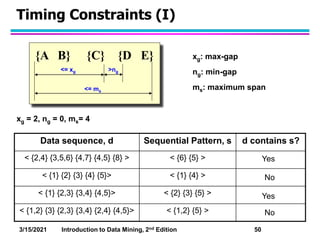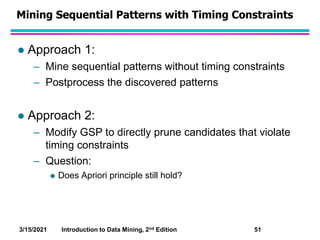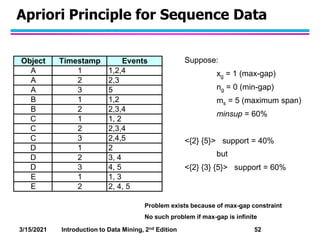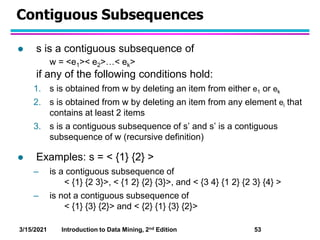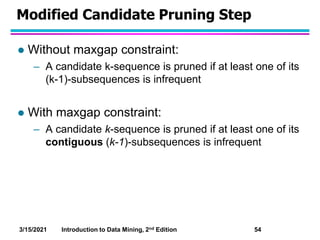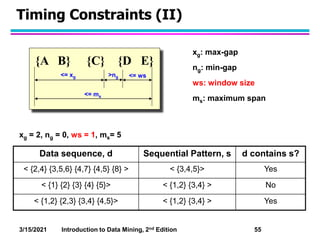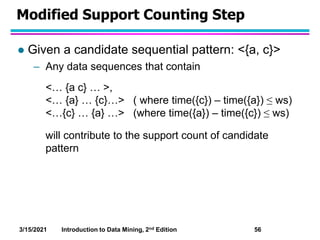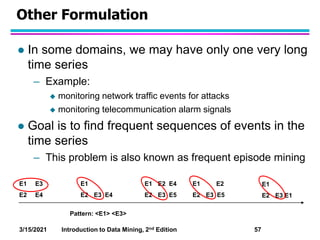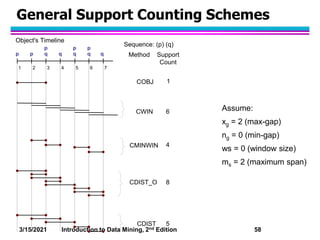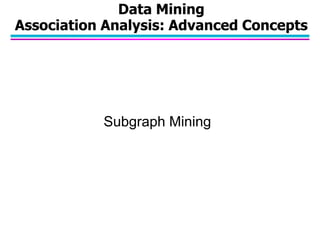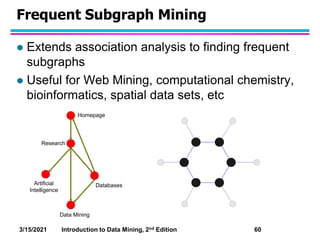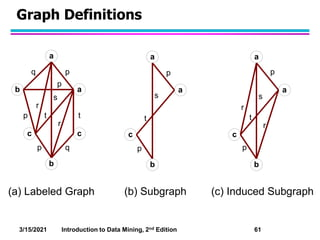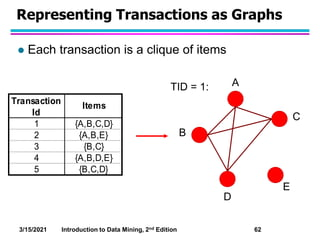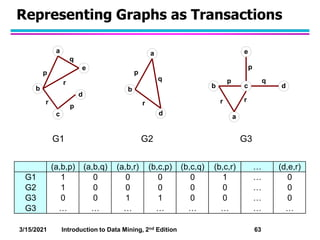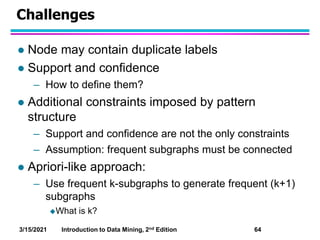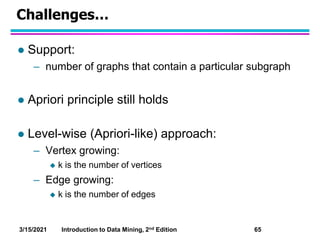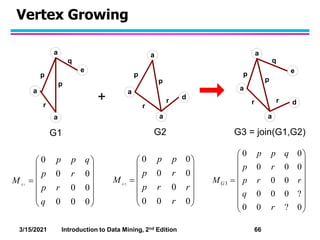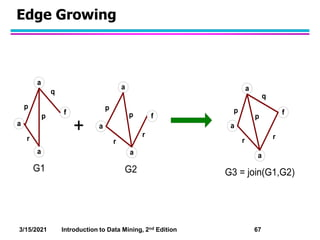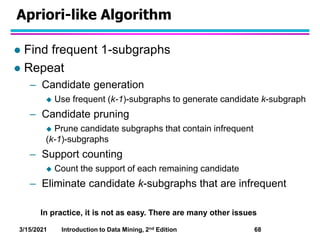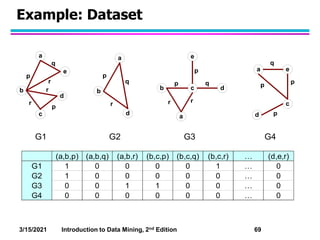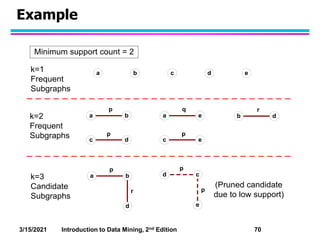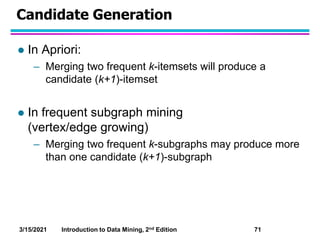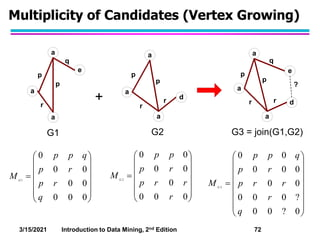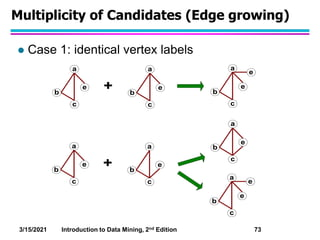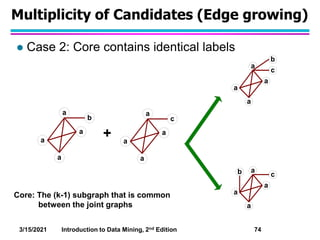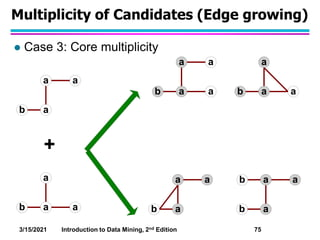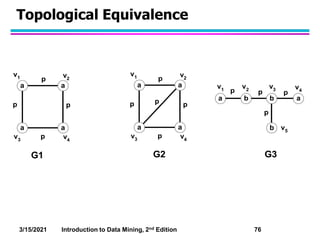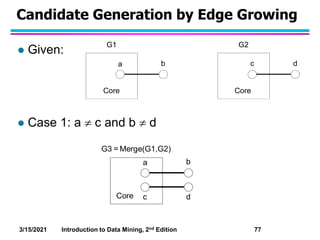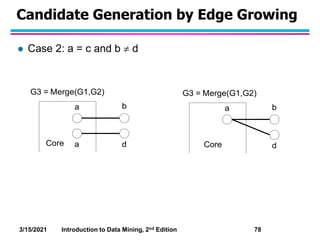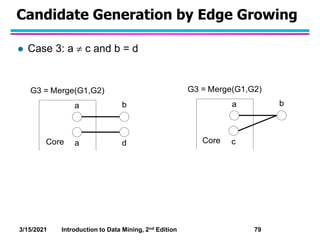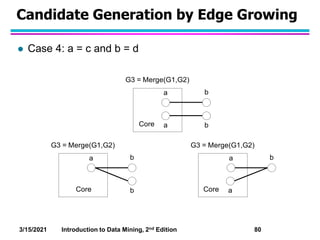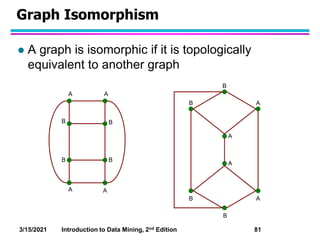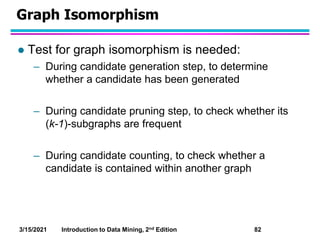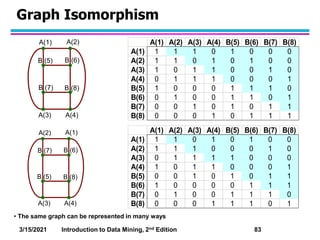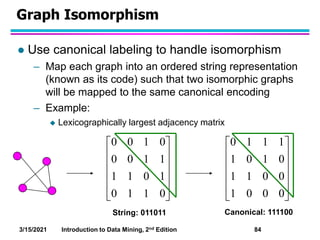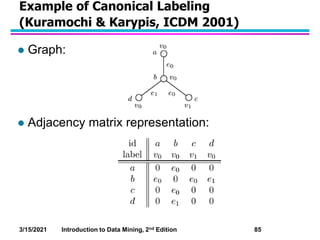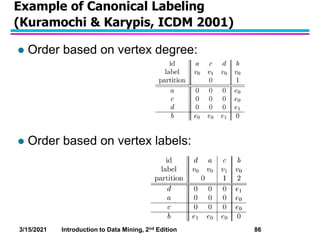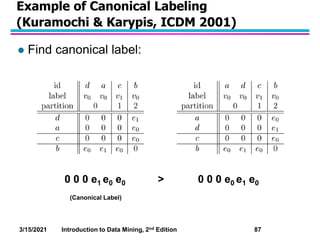1 sur 87

• 1. Chapter 6 Association Analysis: Advance Concepts Introduction to Data Mining, 2nd Edition by Tan, Steinbach, Karpatne, Kumar Data Mining
• 2. Extensions of Association Analysis to Continuous and Categorical Attributes and Multi-level Rules Data Mining Association Analysis: Advanced Concepts
• 3. 3/15/2021 Introduction to Data Mining, 2nd Edition 3 Continuous and Categorical Attributes Example of Association Rule: {Gender=Male, Age  [21,30)}  {No of hours online  10} How to apply association analysis to non-asymmetric binary variables?
• 4. 3/15/2021 Introduction to Data Mining, 2nd Edition 4 Handling Categorical Attributes  Example: Internet Usage Data {Level of Education=Graduate, Online Banking=Yes}  {Privacy Concerns = Yes}
• 5. 3/15/2021 Introduction to Data Mining, 2nd Edition 5 Handling Categorical Attributes  Introduce a new “item” for each distinct attribute- value pair
• 6. 3/15/2021 Introduction to Data Mining, 2nd Edition 6 Handling Categorical Attributes  Some attributes can have many possible values – Many of their attribute values have very low support  Potential solution: Aggregate the low-support attribute values
• 7. 3/15/2021 Introduction to Data Mining, 2nd Edition 7 Handling Categorical Attributes  Distribution of attribute values can be highly skewed – Example: 85% of survey participants own a computer at home  Most records have Computer at home = Yes  Computation becomes expensive; many frequent itemsets involving the binary item (Computer at home = Yes)  Potential solution: – discard the highly frequent items – Use alternative measures such as h-confidence  Computational Complexity – Binarizing the data increases the number of items – But the width of the “transactions” remain the same as the number of original (non-binarized) attributes – Produce more frequent itemsets but maximum size of frequent itemset is limited to the number of original attributes
• 8. 3/15/2021 Introduction to Data Mining, 2nd Edition 8 Handling Continuous Attributes  Different methods: – Discretization-based – Statistics-based – Non-discretization based  minApriori  Different kinds of rules can be produced: – {Age[21,30), No of hours online[10,20)}  {Chat Online =Yes} – {Age[15,30), Covid-Positive = Yes}  Full_recovery
• 9. 3/15/2021 Introduction to Data Mining, 2nd Edition 9 Discretization-based Methods
• 10. 3/15/2021 Introduction to Data Mining, 2nd Edition 10 Discretization-based Methods  Unsupervised: – Equal-width binning – Equal-depth binning – Cluster-based  Supervised discretization 100 150 100 100 0 0 0 100 150 0 0 0 0 20 10 20 0 0 9 8 7 6 5 4 3 2 1 Chat Online = No Chat Online = Yes bin1 bin3 bin2 Continuous attribute, v <1 2 3> <4 5 6> <7 8 9> <1 2 > <3 4 5 6 7 > < 8 9>
• 11. 3/15/2021 Introduction to Data Mining, 2nd Edition 11 Discretization Issues  Interval width 20 40 60 10 30 50 70 20 40 60 10 30 50 70 10 40 70 Age Age Age (a) Original Data (b) Bin = 30 years (c) Bin = 2 years Pattern A Pattern B Pattern C High support region
• 12. 3/15/2021 Introduction to Data Mining, 2nd Edition 12 Discretization Issues  Interval too wide (e.g., Bin size= 30) – May merge several disparate patterns  Patterns A and B are merged together – May lose some of the interesting patterns  Pattern C may not have enough confidence  Interval too narrow (e.g., Bin size = 2) – Pattern A is broken up into two smaller patterns  Can recover the pattern by merging adjacent subpatterns – Pattern B is broken up into smaller patterns  Cannot recover the pattern by merging adjacent subpatterns – Some windows may not meet support threshold
• 13. 3/15/2021 Introduction to Data Mining, 2nd Edition 13 Discretization: all possible intervals  Execution time – If the range is partitioned into k intervals, there are O(k2) new items – If an interval [a,b) is frequent, then all intervals that subsume [a,b) must also be frequent  E.g.: if {Age [21,25), Chat Online=Yes} is frequent, then {Age [10,50), Chat Online=Yes} is also frequent – Improve efficiency:  Use maximum support to avoid intervals that are too wide Number of intervals = k Total number of Adjacent intervals = k(k-1)/2
• 14. 3/15/2021 Introduction to Data Mining, 2nd Edition 14 Statistics-based Methods  Example: {Income > 100K, Online Banking=Yes}  Age: =34  Rule consequent consists of a continuous variable, characterized by their statistics – mean, median, standard deviation, etc.  Approach: – Withhold the target attribute from the rest of the data – Extract frequent itemsets from the rest of the attributes  Binarize the continuous attributes (except for the target attribute) – For each frequent itemset, compute the corresponding descriptive statistics of the target attribute  Frequent itemset becomes a rule by introducing the target variable as rule consequent – Apply statistical test to determine interestingness of the rule
• 15. 3/15/2021 Introduction to Data Mining, 2nd Edition 15 Statistics-based Methods {Male, Income > 100K} {Income < 30K, No hours [10,15)} {Income > 100K, Online Banking = Yes} …. Frequent Itemsets: {Male, Income > 100K}  Age:  = 30 {Income < 40K, No hours [10,15)}  Age:  = 24 {Income > 100K,Online Banking = Yes}  Age:  = 34 …. Association Rules:
• 16. 3/15/2021 Introduction to Data Mining, 2nd Edition 16 Statistics-based Methods  How to determine whether an association rule interesting? – Compare the statistics for segment of population covered by the rule vs segment of population not covered by the rule: A  B:  versus A  B: ’ – Statistical hypothesis testing:  Null hypothesis: H0: ’ =  +   Alternative hypothesis: H1: ’ >  +   Z has zero mean and variance 1 under null hypothesis 2 2 2 1 2 1 ' n s n s Z       
• 17. 3/15/2021 Introduction to Data Mining, 2nd Edition 17 Statistics-based Methods  Example: r: Covid-Postive & Quick_Recovery=Yes  Age: =23 – Rule is interesting if difference between  and ’ is more than 5 years (i.e.,  = 5) – For r, suppose n1 = 50, s1 = 3.5 – For r’ (complement): n2 = 250, s2 = 6.5 – For 1-sided test at 95% confidence level, critical Z-value for rejecting null hypothesis is 1.64. – Since Z is greater than 1.64, r is an interesting rule 11 . 3 250 5 . 6 50 5 . 3 5 23 30 ' 2 2 2 2 2 1 2 1           n s n s Z  
• 18. 3/15/2021 Introduction to Data Mining, 2nd Edition 18 Min-Apriori TID W1 W2 W3 W4 W5 D1 2 2 0 0 1 D2 0 0 1 2 2 D3 2 3 0 0 0 D4 0 0 1 0 1 D5 1 1 1 0 2 Example: W1 and W2 tends to appear together in the same document Document-term matrix:
• 19. 3/15/2021 Introduction to Data Mining, 2nd Edition 19 Min-Apriori  Data contains only continuous attributes of the same “type” – e.g., frequency of words in a document  Potential solution: – Convert into 0/1 matrix and then apply existing algorithms  lose word frequency information – Discretization does not apply as users want association among words based on how frequently they co-occur, not if they occur with similar frequencies TID W1 W2 W3 W4 W5 D1 2 2 0 0 1 D2 0 0 1 2 2 D3 2 3 0 0 0 D4 0 0 1 0 1 D5 1 1 1 0 2
• 20. 3/15/2021 Introduction to Data Mining, 2nd Edition 20 Min-Apriori  How to determine the support of a word? – If we simply sum up its frequency, support count will be greater than total number of documents!  Normalize the word vectors – e.g., using L1 norms  Each word has a support equals to 1.0 TID W1 W2 W3 W4 W5 D1 2 2 0 0 1 D2 0 0 1 2 2 D3 2 3 0 0 0 D4 0 0 1 0 1 D5 1 1 1 0 2 TID W1 W2 W3 W4 W5 D1 0.40 0.33 0.00 0.00 0.17 D2 0.00 0.00 0.33 1.00 0.33 D3 0.40 0.50 0.00 0.00 0.00 D4 0.00 0.00 0.33 0.00 0.17 D5 0.20 0.17 0.33 0.00 0.33 Normalize
• 21. 3/15/2021 Introduction to Data Mining, 2nd Edition 21 Min-Apriori  New definition of support:     T i C j j i D C ) , ( ) sup( min Example: Sup(W1,W2) = .33 + 0 + .4 + 0 + 0.17 = 0.9 TID W1 W2 W3 W4 W5 D1 0.40 0.33 0.00 0.00 0.17 D2 0.00 0.00 0.33 1.00 0.33 D3 0.40 0.50 0.00 0.00 0.00 D4 0.00 0.00 0.33 0.00 0.17 D5 0.20 0.17 0.33 0.00 0.33
• 22. 3/15/2021 Introduction to Data Mining, 2nd Edition 22 Anti-monotone property of Support Example: Sup(W1) = 0.4 + 0 + 0.4 + 0 + 0.2 = 1 Sup(W1, W2) = 0.33 + 0 + 0.4 + 0 + 0.17 = 0.9 Sup(W1, W2, W3) = 0 + 0 + 0 + 0 + 0.17 = 0.17 TID W1 W2 W3 W4 W5 D1 0.40 0.33 0.00 0.00 0.17 D2 0.00 0.00 0.33 1.00 0.33 D3 0.40 0.50 0.00 0.00 0.00 D4 0.00 0.00 0.33 0.00 0.17 D5 0.20 0.17 0.33 0.00 0.33
• 23. 3/15/2021 Introduction to Data Mining, 2nd Edition 23 Concept Hierarchies Food Bread Milk Skim 2% Electronics Computers Home Desktop Laptop Wheat White Foremost Kemps DVD TV Printer Scanner Accessory
• 24. 3/15/2021 Introduction to Data Mining, 2nd Edition 24 Multi-level Association Rules  Why should we incorporate concept hierarchy? – Rules at lower levels may not have enough support to appear in any frequent itemsets – Rules at lower levels of the hierarchy are overly specific  e.g., following rules are indicative of association between milk and bread – skim milk  white bread, – 2% milk  wheat bread, – skim milk  wheat bread, etc. – Rules at higher level of hierarchy may be too generic  e.g., electronics  food
• 25. 3/15/2021 Introduction to Data Mining, 2nd Edition 25 Multi-level Association Rules  How do support and confidence vary as we traverse the concept hierarchy? – If (X1  Y1) ≥ minsup, and X is parent of X1, Y is parent of Y1 then (X  Y1) ≥ minsup, (X1  Y) ≥ minsup (X  Y) ≥ minsup – If conf(X1  Y1) ≥ minconf, then conf(X1  Y) ≥ minconf
• 26. 3/15/2021 Introduction to Data Mining, 2nd Edition 26 Multi-level Association Rules  Approach 1: – Extend current association rule formulation by augmenting each transaction with higher level items Original Transaction: {skim milk, wheat bread} Augmented Transaction: {skim milk, wheat bread, milk, bread, food}  Issues: – Items that reside at higher levels have much higher support counts  if support threshold is low, too many frequent patterns involving items from the higher levels – Increased dimensionality of the data
• 27. 3/15/2021 Introduction to Data Mining, 2nd Edition 27 Multi-level Association Rules  Approach 2: – Generate frequent patterns at highest level first – Then, generate frequent patterns at the next highest level, and so on  Issues: – I/O requirements will increase dramatically because we need to perform more passes over the data – May miss some potentially interesting cross-level association patterns
• 28. Sequential Patterns Data Mining Association Analysis: Advanced Concepts
• 29. 3/15/2021 Introduction to Data Mining, 2nd Edition 29 Examples of Sequence  Sequence of different transactions by a customer at an online store: < {Digital Camera,iPad} {memory card} {headphone,iPad cover} >  Sequence of initiating events causing the nuclear accident at 3-mile Island: (http://stellar-one.com/nuclear/staff_reports/summary_SOE_the_initiating_event.htm) < {clogged resin} {outlet valve closure} {loss of feedwater} {condenser polisher outlet valve shut} {booster pumps trip} {main waterpump trips} {main turbine trips} {reactor pressure increases}>  Sequence of books checked out at a library: <{Fellowship of the Ring} {The Two Towers} {Return of the King}>
• 30. 3/15/2021 Introduction to Data Mining, 2nd Edition 30 Sequential Pattern Discovery: Examples  In telecommunications alarm logs, – Inverter_Problem: (Excessive_Line_Current) (Rectifier_Alarm) --> (Fire_Alarm)  In point-of-sale transaction sequences, – Computer Bookstore: (Intro_To_Visual_C) (C++_Primer) --> (Perl_for_dummies,Tcl_Tk) – Athletic Apparel Store: (Shoes) (Racket, Racketball) --> (Sports_Jacket)
• 31. 3/15/2021 Introduction to Data Mining, 2nd Edition 31 Sequence Data Sequence Database Sequence Element (Transaction) Event (Item) Customer Purchase history of a given customer A set of items bought by a customer at time t Books, diary products, CDs, etc Web Data Browsing activity of a particular Web visitor A collection of files viewed by a Web visitor after a single mouse click Home page, index page, contact info, etc Event data History of events generated by a given sensor Events triggered by a sensor at time t Types of alarms generated by sensors Genome sequences DNA sequence of a particular species An element of the DNA sequence Bases A,T,G,C Sequence E1 E2 E1 E3 E2 E3 E4 E2 Element (Transaction) Event (Item)
• 32. 3/15/2021 Introduction to Data Mining, 2nd Edition 32 Sequence Data 10 15 20 25 30 35 2 3 5 6 1 1 Timeline Object A: Object B: Object C: 4 5 6 2 7 8 1 2 1 6 1 7 8 Sequence ID Timestamp Events A 10 2, 3, 5 A 20 6, 1 A 23 1 B 11 4, 5, 6 B 17 2 B 21 7, 8, 1, 2 B 28 1, 6 C 14 1, 8, 7 Sequence Database: Sequence A: Sequence B: Sequence C:
• 33. 3/15/2021 Introduction to Data Mining, 2nd Edition 33 Sequence Data vs. Market-basket Data Customer Date Items bought A 10 2, 3, 5 A 20 1,6 A 23 1 B 11 4, 5, 6 B 17 2 B 21 1,2,7,8 B 28 1, 6 C 14 1,7,8 Sequence Database: Events 2, 3, 5 1,6 1 4,5,6 2 1,2,7,8 1,6 1,7,8 Market- basket Data
• 34. 3/15/2021 Introduction to Data Mining, 2nd Edition 34 Sequence Data vs. Market-basket Data Customer Date Items bought A 10 2, 3, 5 A 20 1,6 A 23 1 B 11 4, 5, 6 B 17 2 B 21 1,2,7,8 B 28 1, 6 C 14 1,7,8 Sequence Database: Events 2, 3, 5 1,6 1 4,5,6 2 1,2,7,8 1,6 1,7,8 Market- basket Data
• 35. 3/15/2021 Introduction to Data Mining, 2nd Edition 35 Formal Definition of a Sequence  A sequence is an ordered list of elements s = < e1 e2 e3 … > – Each element contains a collection of events (items) ei = {i1, i2, …, ik}  Length of a sequence, |s|, is given by the number of elements in the sequence  A k-sequence is a sequence that contains k events (items) – <{a,b} {a}> has a length of 2 and it is a 3-sequence
• 36. 3/15/2021 Introduction to Data Mining, 2nd Edition 36 Formal Definition of a Subsequence  A sequence t: <a1 a2 … an> is contained in another sequence s: <b1 b2 … bm> (m ≥ n) if there exist integers i1 < i2 < … < in such that a1  bi1 , a2  bi2, …, an  bin  Illustrative Example: s: b1 b2 b3 b4 b5 t: a1 a2 a3 t is a subsequence of s if a1 b2, a2 b3, a3 b5. Data sequence Subsequence Contain? < {2,4} {3,5,6} {8} > < {2} {8} > < {1,2} {3,4} > < {1} {2} > < {2,4} {2,4} {2,5} > < {2} {4} > <{2,4} {2,5} {4,5}> < {2} {4} {5} > <{2,4} {2,5} {4,5}> < {2} {5} {5} > <{2,4} {2,5} {4,5}> < {2, 4, 5} > No Yes Yes Yes No No
• 37. 3/15/2021 Introduction to Data Mining, 2nd Edition 37 Sequential Pattern Mining: Definition  The support of a subsequence w is defined as the fraction of data sequences that contain w  A sequential pattern is a frequent subsequence (i.e., a subsequence whose support is ≥ minsup)  Given: – a database of sequences – a user-specified minimum support threshold, minsup  Task: – Find all subsequences with support ≥ minsup
• 38. 3/15/2021 Introduction to Data Mining, 2nd Edition 38 Sequential Pattern Mining: Example Minsup = 50% Examples of Frequent Subsequences: < {1,2} > s=60% < {2,3} > s=60% < {2,4}> s=80% < {3} {5}> s=80% < {1} {2} > s=80% < {2} {2} > s=60% < {1} {2,3} > s=60% < {2} {2,3} > s=60% < {1,2} {2,3} > s=60% Object Timestamp Events A 1 1,2,4 A 2 2,3 A 3 5 B 1 1,2 B 2 2,3,4 C 1 1, 2 C 2 2,3,4 C 3 2,4,5 D 1 2 D 2 3, 4 D 3 4, 5 E 1 1, 3 E 2 2, 4, 5
• 39. 3/15/2021 Introduction to Data Mining, 2nd Edition 39 Sequence Data vs. Market-basket Data Customer Date Items bought A 10 2, 3, 5 A 20 1,6 A 23 1 B 11 4, 5, 6 B 17 2 B 21 1,2,7,8 B 28 1, 6 C 14 1,7,8 Sequence Database: Events 2, 3, 5 1,6 1 4,5,6 2 1,2,7,8 1,6 1,7,8 Market- basket Data (1,8) -> (7) {2} -> {1}
• 40. 3/15/2021 Introduction to Data Mining, 2nd Edition 40 Extracting Sequential Patterns  Given n events: i1, i2, i3, …, in  Candidate 1-subsequences: <{i1}>, <{i2}>, <{i3}>, …, <{in}>  Candidate 2-subsequences: <{i1, i2}>, <{i1, i3}>, …, <{i1} {i1}>, <{i1} {i2}>, …, <{in} {in}>  Candidate 3-subsequences: <{i1, i2 , i3}>, <{i1, i2 , i4}>, …, <{i1, i2} {i1}>, <{i1, i2} {i2}>, …, <{i1} {i1 , i2}>, <{i1} {i1 , i3}>, …, <{i1} {i1} {i1}>, <{i1} {i1} {i2}>, …
• 41. 3/15/2021 Introduction to Data Mining, 2nd Edition 41 Extracting Sequential Patterns: Simple example  Given 2 events: a, b  Candidate 1-subsequences: <{a}>, <{b}>.  Candidate 2-subsequences: <{a} {a}>, <{a} {b}>, <{b} {a}>, <{b} {b}>, <{a, b}>.  Candidate 3-subsequences: <{a} {a} {a}>, <{a} {a} {b}>, <{a} {b} {a}>, <{a} {b} {b}>, <{b} {b} {b}>, <{b} {b} {a}>, <{b} {a} {b}>, <{b} {a} {a}> <{a, b} {a}>, <{a, b} {b}>, <{a} {a, b}>, <{b} {a, b}> () (a) (b) (a,b) Item-set patterns
• 42. 3/15/2021 Introduction to Data Mining, 2nd Edition 42 Generalized Sequential Pattern (GSP)  Step 1: – Make the first pass over the sequence database D to yield all the 1- element frequent sequences  Step 2: Repeat until no new frequent sequences are found – Candidate Generation:  Merge pairs of frequent subsequences found in the (k-1)th pass to generate candidate sequences that contain k items – Candidate Pruning:  Prune candidate k-sequences that contain infrequent (k-1)-subsequences – Support Counting:  Make a new pass over the sequence database D to find the support for these candidate sequences – Candidate Elimination:  Eliminate candidate k-sequences whose actual support is less than minsup
• 43. 3/15/2021 Introduction to Data Mining, 2nd Edition 43 Candidate Generation  Base case (k=2): – Merging two frequent 1-sequences <{i1}> and <{i2}> will produce the following candidate 2-sequences: <{i1} {i1}>, <{i1} {i2}>, <{i2} {i2}>, <{i2} {i1}> and <{i1, i2}>. (Note: <{i1}> can be merged with itself to produce: <{i1} {i1}>)  General case (k>2): – A frequent (k-1)-sequence w1 is merged with another frequent (k-1)-sequence w2 to produce a candidate k-sequence if the subsequence obtained by removing an event from the first element in w1 is the same as the subsequence obtained by removing an event from the last element in w2
• 44. 3/15/2021 Introduction to Data Mining, 2nd Edition 44 Candidate Generation  Base case (k=2): – Merging two frequent 1-sequences <{i1}> and <{i2}> will produce the following candidate 2-sequences: <{i1} {i1}>, <{i1} {i2}>, <{i2} {i2}>, <{i2} {i1}> and <{i1 i2}>. (Note: <{i1}> can be merged with itself to produce: <{i1} {i1}>)  General case (k>2): – A frequent (k-1)-sequence w1 is merged with another frequent (k-1)-sequence w2 to produce a candidate k-sequence if the subsequence obtained by removing an event from the first element in w1 is the same as the subsequence obtained by removing an event from the last element in w2  The resulting candidate after merging is given by extending the sequence w1 as follows- – If the last element of w2 has only one event, append it to w1 – Otherwise add the event from the last element of w2 (which is absent in the last element of w1) to the last element of w1
• 45. 11/19/2012 Introduction to Data Mining 45 Candidate Generation Examples  Merging w1=<{1 2 3} {4 6}> and w2 =<{2 3} {4 6} {5}> produces the candidate sequence < {1 2 3} {4 6} {5}> because the last element of w2 has only one event  Merging w1=<{1} {2 3} {4}> and w2 =<{2 3} {4 5}> produces the candidate sequence < {1} {2 3} {4 5}> because the last element in w2 has more than one event  Merging w1=<{1 2 3} > and w2 =<{2 3 4} > produces the candidate sequence < {1 2 3 4}> because the last element in w2 has more than one event  We do not have to merge the sequences w1 =<{1} {2 6} {4}> and w2 =<{1} {2} {4 5}> to produce the candidate < {1} {2 6} {4 5}> because if the latter is a viable candidate, then it can be obtained by merging w1 with < {2 6} {4 5}>
• 46. 11/19/2012 Introduction to Data Mining 46 Candidate Generation: Examples (ctd)  Can <{a},{b},{c}> merge with <{b},{c},{f}> ?  Can <{a},{b},{c}> merge with <{b,c},{f}>?  Can <{a},{b},{c}> merge with <{b},{c,f}>?  Can <{a,b},{c}> merge with <{b},{c,f}> ?  Can <{a,b,c}> merge with <{b,c,f}>?  Can <{a}> merge with <{a}>?
• 47. 11/19/2012 Introduction to Data Mining 47 Candidate Generation: Examples (ctd)  <{a},{b},{c}> can be merged with <{b},{c},{f}> to produce <{a},{b},{c},{f}>  <{a},{b},{c}> cannot be merged with <{b,c},{f}>  <{a},{b},{c}> can be merged with <{b},{c,f}> to produce <{a},{b},{c,f}>  <{a,b},{c}> can be merged with <{b},{c,f}> to produce <{a,b},{c,f}>  <{a,b,c}> can be merged with <{b,c,f}> to produce <{a,b,c,f}>  <{a}{b}{a}> can be merged with <{b}{a}{b}> to produce <{a},{b},{a},{b}>  <{b}{a}{b}> can be merged with <{a}{b}{a}> to produce <{b},{a},{b},{a}>
• 48. 11/19/2012 Introduction to Data Mining 48 GSP Example < {1} {2} {3} > < {1} {2 5} > < {1} {5} {3} > < {2} {3} {4} > < {2 5} {3} > < {3} {4} {5} > < {5} {3 4} > Frequent 3-sequences < {1} {2} {3} {4} > < {1} {2 5} {3} > < {1} {5} {3 4} > < {2} {3} {4} {5} > < {2 5} {3 4} > Candidate Generation
• 49. 11/19/2012 Introduction to Data Mining 49 GSP Example < {1} {2} {3} > < {1} {2 5} > < {1} {5} {3} > < {2} {3} {4} > < {2 5} {3} > < {3} {4} {5} > < {5} {3 4} > Frequent 3-sequences < {1} {2} {3} {4} > < {1} {2 5} {3} > < {1} {5} {3 4} > < {2} {3} {4} {5} > < {2 5} {3 4} > Candidate Generation < {1} {2 5} {3} > Candidate Pruning
• 50. 3/15/2021 Introduction to Data Mining, 2nd Edition 50 Timing Constraints (I) {A B} {C} {D E} <= ms <= xg >ng xg: max-gap ng: min-gap ms: maximum span Data sequence, d Sequential Pattern, s d contains s? < {2,4} {3,5,6} {4,7} {4,5} {8} > < {6} {5} > < {1} {2} {3} {4} {5}> < {1} {4} > < {1} {2,3} {3,4} {4,5}> < {2} {3} {5} > < {1,2} {3} {2,3} {3,4} {2,4} {4,5}> < {1,2} {5} > xg = 2, ng = 0, ms= 4 Yes Yes No No
• 51. 3/15/2021 Introduction to Data Mining, 2nd Edition 51 Mining Sequential Patterns with Timing Constraints  Approach 1: – Mine sequential patterns without timing constraints – Postprocess the discovered patterns  Approach 2: – Modify GSP to directly prune candidates that violate timing constraints – Question:  Does Apriori principle still hold?
• 52. 3/15/2021 Introduction to Data Mining, 2nd Edition 52 Apriori Principle for Sequence Data Object Timestamp Events A 1 1,2,4 A 2 2,3 A 3 5 B 1 1,2 B 2 2,3,4 C 1 1, 2 C 2 2,3,4 C 3 2,4,5 D 1 2 D 2 3, 4 D 3 4, 5 E 1 1, 3 E 2 2, 4, 5 Suppose: xg = 1 (max-gap) ng = 0 (min-gap) ms = 5 (maximum span) minsup = 60% <{2} {5}> support = 40% Problem exists because of max-gap constraint No such problem if max-gap is infinite but <{2} {3} {5}> support = 60%
• 53. 3/15/2021 Introduction to Data Mining, 2nd Edition 53 Contiguous Subsequences  s is a contiguous subsequence of w = <e1>< e2>…< ek> if any of the following conditions hold: 1. s is obtained from w by deleting an item from either e1 or ek 2. s is obtained from w by deleting an item from any element ei that contains at least 2 items 3. s is a contiguous subsequence of s’ and s’ is a contiguous subsequence of w (recursive definition)  Examples: s = < {1} {2} > – is a contiguous subsequence of < {1} {2 3}>, < {1 2} {2} {3}>, and < {3 4} {1 2} {2 3} {4} > – is not a contiguous subsequence of < {1} {3} {2}> and < {2} {1} {3} {2}>
• 54. 3/15/2021 Introduction to Data Mining, 2nd Edition 54 Modified Candidate Pruning Step  Without maxgap constraint: – A candidate k-sequence is pruned if at least one of its (k-1)-subsequences is infrequent  With maxgap constraint: – A candidate k-sequence is pruned if at least one of its contiguous (k-1)-subsequences is infrequent
• 55. 3/15/2021 Introduction to Data Mining, 2nd Edition 55 Timing Constraints (II) {A B} {C} {D E} <= ms <= xg >ng <= ws xg: max-gap ng: min-gap ws: window size ms: maximum span Data sequence, d Sequential Pattern, s d contains s? < {2,4} {3,5,6} {4,7} {4,5} {8} > < {3,4,5}> Yes < {1} {2} {3} {4} {5}> < {1,2} {3,4} > No < {1,2} {2,3} {3,4} {4,5}> < {1,2} {3,4} > Yes xg = 2, ng = 0, ws = 1, ms= 5
• 56. 3/15/2021 Introduction to Data Mining, 2nd Edition 56 Modified Support Counting Step  Given a candidate sequential pattern: <{a, c}> – Any data sequences that contain <… {a c} … >, <… {a} … {c}…> ( where time({c}) – time({a}) ≤ ws) <…{c} … {a} …> (where time({a}) – time({c}) ≤ ws) will contribute to the support count of candidate pattern
• 57. 3/15/2021 Introduction to Data Mining, 2nd Edition 57 Other Formulation  In some domains, we may have only one very long time series – Example:  monitoring network traffic events for attacks  monitoring telecommunication alarm signals  Goal is to find frequent sequences of events in the time series – This problem is also known as frequent episode mining E1 E2 E1 E2 E1 E2 E3 E4 E3 E4 E1 E2 E2 E4 E3 E5 E2 E3 E5 E1 E2 E3 E1 Pattern: <E1> <E3>
• 58. 3/15/2021 Introduction to Data Mining, 2nd Edition 58 General Support Counting Schemes p Object's Timeline Sequence: (p) (q) Method Support Count COBJ 1 1 CWIN 6 CMINWIN 4 p q p q q p q q p 2 3 4 5 6 7 CDIST_O 8 CDIST 5 Assume: xg = 2 (max-gap) ng = 0 (min-gap) ws = 0 (window size) ms = 2 (maximum span)
• 59. Subgraph Mining Data Mining Association Analysis: Advanced Concepts
• 60. 3/15/2021 Introduction to Data Mining, 2nd Edition 60 Frequent Subgraph Mining  Extends association analysis to finding frequent subgraphs  Useful for Web Mining, computational chemistry, bioinformatics, spatial data sets, etc Databases Homepage Research Artificial Intelligence Data Mining
• 61. 3/15/2021 Introduction to Data Mining, 2nd Edition 61 Graph Definitions a b a c c b (a) Labeled Graph p q p p r s t r t q p a a c b (b) Subgraph p s t p a a c b (c) Induced Subgraph p r s t r p
• 62. 3/15/2021 Introduction to Data Mining, 2nd Edition 62 Representing Transactions as Graphs  Each transaction is a clique of items Transaction Id Items 1 {A,B,C,D} 2 {A,B,E} 3 {B,C} 4 {A,B,D,E} 5 {B,C,D} A B C D E TID = 1:
• 63. 3/15/2021 Introduction to Data Mining, 2nd Edition 63 Representing Graphs as Transactions a b e c p q r p a b d p r G1 G2 q e c a p q r b p G3 d r d r (a,b,p) (a,b,q) (a,b,r) (b,c,p) (b,c,q) (b,c,r) … (d,e,r) G1 1 0 0 0 0 1 … 0 G2 1 0 0 0 0 0 … 0 G3 0 0 1 1 0 0 … 0 G3 … … … … … … … …
• 64. 3/15/2021 Introduction to Data Mining, 2nd Edition 64 Challenges  Node may contain duplicate labels  Support and confidence – How to define them?  Additional constraints imposed by pattern structure – Support and confidence are not the only constraints – Assumption: frequent subgraphs must be connected  Apriori-like approach: – Use frequent k-subgraphs to generate frequent (k+1) subgraphs What is k?
• 65. 3/15/2021 Introduction to Data Mining, 2nd Edition 65 Challenges…  Support: – number of graphs that contain a particular subgraph  Apriori principle still holds  Level-wise (Apriori-like) approach: – Vertex growing:  k is the number of vertices – Edge growing:  k is the number of edges
• 66. 3/15/2021 Introduction to Data Mining, 2nd Edition 66 Vertex Growing a a e a p q r p a a a p r r d G1 G2 p                0 0 0 0 0 0 0 0 1 q r p r p q p p MG                0 0 0 0 0 0 0 0 2 r r r p r p p p MG a a a p q r e p                  0 ? 0 0 ? 0 0 0 0 0 0 0 0 0 0 3 r q r r p r p q p p MG G3 = join(G1,G2) d r +
• 67. 3/15/2021 Introduction to Data Mining, 2nd Edition 67 Edge Growing a a f a p q r p a a a p r r f G1 G2 p a a a p q r f p G3 = join(G1,G2) r +
• 68. 3/15/2021 Introduction to Data Mining, 2nd Edition 68 Apriori-like Algorithm  Find frequent 1-subgraphs  Repeat – Candidate generation  Use frequent (k-1)-subgraphs to generate candidate k-subgraph – Candidate pruning  Prune candidate subgraphs that contain infrequent (k-1)-subgraphs – Support counting  Count the support of each remaining candidate – Eliminate candidate k-subgraphs that are infrequent In practice, it is not as easy. There are many other issues
• 69. 3/15/2021 Introduction to Data Mining, 2nd Edition 69 Example: Dataset a b e c p q r p a b d p r G1 G2 q e c a p q r b p G3 d r d r (a,b,p) (a,b,q) (a,b,r) (b,c,p) (b,c,q) (b,c,r) … (d,e,r) G1 1 0 0 0 0 1 … 0 G2 1 0 0 0 0 0 … 0 G3 0 0 1 1 0 0 … 0 G4 0 0 0 0 0 0 … 0 a e q c d p p p G4 r
• 70. 3/15/2021 Introduction to Data Mining, 2nd Edition 70 Example p a b c d e k=1 Frequent Subgraphs a b p c d p c e q a e r b d p a b d r p d c e p (Pruned candidate due to low support) Minimum support count = 2 k=2 Frequent Subgraphs k=3 Candidate Subgraphs
• 71. 3/15/2021 Introduction to Data Mining, 2nd Edition 71 Candidate Generation  In Apriori: – Merging two frequent k-itemsets will produce a candidate (k+1)-itemset  In frequent subgraph mining (vertex/edge growing) – Merging two frequent k-subgraphs may produce more than one candidate (k+1)-subgraph
• 72. 3/15/2021 Introduction to Data Mining, 2nd Edition 72 Multiplicity of Candidates (Vertex Growing) a a e a p q r p a a a p r r d G1 G2 p                0 0 0 0 0 0 0 0 1 q r p r p q p p MG                0 0 0 0 0 0 0 0 2 r r r p r p p p MG a a a p q r e p                  0 ? 0 0 ? 0 0 0 0 0 0 0 0 0 0 3 q r r r p r p q p p M G G3 = join(G1,G2) d r ? +
• 73. 3/15/2021 Introduction to Data Mining, 2nd Edition 73 Multiplicity of Candidates (Edge growing)  Case 1: identical vertex labels a b e c a b e c + a b e c e a b e c a b e c a b e c + e a b e c
• 74. 3/15/2021 Introduction to Data Mining, 2nd Edition 74 Multiplicity of Candidates (Edge growing)  Case 2: Core contains identical labels + a a a a c b a a a a c b a a a a b a a a a c Core: The (k-1) subgraph that is common between the joint graphs
• 75. 3/15/2021 Introduction to Data Mining, 2nd Edition 75 Multiplicity of Candidates (Edge growing)  Case 3: Core multiplicity a a b + a a a a b a a b a a a b a a a b a b a a b a a
• 76. 3/15/2021 Introduction to Data Mining, 2nd Edition 76 Topological Equivalence p a a a a p p p v1 v2 v3 v4 p a a a a p p p v1 v2 v3 v4 p b a b a p G1 p v1 v2 v3 v4 b p p G2 G3 v5
• 77. 3/15/2021 Introduction to Data Mining, 2nd Edition 77 Candidate Generation by Edge Growing  Given:  Case 1: a  c and b  d a b c d G1 G2 Core Core a b c d G3 = Merge(G1,G2) Core
• 78. 3/15/2021 Introduction to Data Mining, 2nd Edition 78 Candidate Generation by Edge Growing  Case 2: a = c and b  d a b a d G3 = Merge(G1,G2) Core a b d G3 = Merge(G1,G2) Core
• 79. 3/15/2021 Introduction to Data Mining, 2nd Edition 79 Candidate Generation by Edge Growing  Case 3: a  c and b = d a b a d G3 = Merge(G1,G2) Core a b G3 = Merge(G1,G2) Core c
• 80. 3/15/2021 Introduction to Data Mining, 2nd Edition 80 Candidate Generation by Edge Growing  Case 4: a = c and b = d a b a b G3 = Merge(G1,G2) Core a b G3 = Merge(G1,G2) Core a a b b G3 = Merge(G1,G2) Core
• 81. 3/15/2021 Introduction to Data Mining, 2nd Edition 81 Graph Isomorphism  A graph is isomorphic if it is topologically equivalent to another graph A A A A B A B A B B A A B B B B
• 82. 3/15/2021 Introduction to Data Mining, 2nd Edition 82 Graph Isomorphism  Test for graph isomorphism is needed: – During candidate generation step, to determine whether a candidate has been generated – During candidate pruning step, to check whether its (k-1)-subgraphs are frequent – During candidate counting, to check whether a candidate is contained within another graph
• 83. 3/15/2021 Introduction to Data Mining, 2nd Edition 83 Graph Isomorphism A(1) A(2) B (6) A(4) B (5) A(3) B (7) B (8) A(1) A(2) A(3) A(4) B(5) B(6) B(7) B(8) A(1) 1 1 1 0 1 0 0 0 A(2) 1 1 0 1 0 1 0 0 A(3) 1 0 1 1 0 0 1 0 A(4) 0 1 1 1 0 0 0 1 B(5) 1 0 0 0 1 1 1 0 B(6) 0 1 0 0 1 1 0 1 B(7) 0 0 1 0 1 0 1 1 B(8) 0 0 0 1 0 1 1 1 A(2) A(1) B (6) A(4) B (7) A(3) B (5) B (8) A(1) A(2) A(3) A(4) B(5) B(6) B(7) B(8) A(1) 1 1 0 1 0 1 0 0 A(2) 1 1 1 0 0 0 1 0 A(3) 0 1 1 1 1 0 0 0 A(4) 1 0 1 1 0 0 0 1 B(5) 0 0 1 0 1 0 1 1 B(6) 1 0 0 0 0 1 1 1 B(7) 0 1 0 0 1 1 1 0 B(8) 0 0 0 1 1 1 0 1 • The same graph can be represented in many ways
• 84. 3/15/2021 Introduction to Data Mining, 2nd Edition 84 Graph Isomorphism  Use canonical labeling to handle isomorphism – Map each graph into an ordered string representation (known as its code) such that two isomorphic graphs will be mapped to the same canonical encoding – Example:  Lexicographically largest adjacency matrix             0 1 1 0 1 0 1 1 1 1 0 0 0 1 0 0 String: 011011             0 0 0 1 0 0 1 1 0 1 0 1 1 1 1 0 Canonical: 111100
• 85. 3/15/2021 Introduction to Data Mining, 2nd Edition 85 Example of Canonical Labeling (Kuramochi & Karypis, ICDM 2001)  Graph:  Adjacency matrix representation:
• 86. 3/15/2021 Introduction to Data Mining, 2nd Edition 86 Example of Canonical Labeling (Kuramochi & Karypis, ICDM 2001)  Order based on vertex degree:  Order based on vertex labels:
• 87. 3/15/2021 Introduction to Data Mining, 2nd Edition 87 Example of Canonical Labeling (Kuramochi & Karypis, ICDM 2001)  Find canonical label: 0 0 0 e1 e0 e0 0 0 0 e0 e1 e0 > (Canonical Label)